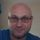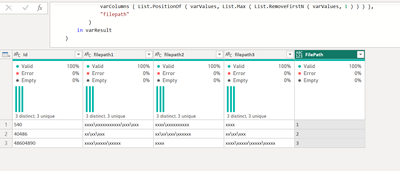cancel
Showing results for
Did you mean:Helper V

## retain longest value of a group of columns for each row

Hello,

I have some data which looks something like this:

 filepath1 filepath2 filepath3 540 xxxx\xxxxxxxxxxx\xxx\xxx xxxx\xxxxxxxxxx xxxx 40486 xx\xx\xxx xx\xx\xxx\xxxxxx xx\xx\xxx 48604890 xxxx\xxxxx\xxxxx xxxx xxxx\xxxxx\xxxxx\xxxxx

and I need to keep, for each row, the path with the maximum length.

So, in this case, it would be:

 id filepath 540 1 40486 2 48604890 3

Any ideas?

1 ACCEPTED SOLUTIONSuper User

The _ is just a reference to the current row's values.  Please try this instead.

``````let
Source = Table.FromRows(Json.Document(Binary.Decompress(Binary.FromText("i45WMjUxUNJRqgCCmJgKBABzwAQWWaiQUqxOtJKJgYmFGVgApAKhA86DaURTA9ZrYQbUbYnmACgFswSbHExJbCwA", BinaryEncoding.Base64), Compression.Deflate)), let _t = ((type nullable text) meta [Serialized.Text = true]) in type table [Id = _t, filepath1 = _t, filepath2 = _t, filepath3 = _t]),
let varColumns = List.Select ( Table.ColumnNames ( Source ), each Text.Contains ( _, "filepath" ) ) in
each
let
varValues = Record.FieldValues ( Record.SelectFields ( _ , varColumns ) ),
varResult = Text.AfterDelimiter (
varColumns { List.PositionOf ( varValues, List.Max ( varValues ) ) },
"filepath"
)
in varResult
)
in
7 REPLIES 7Helper V

Thank you. Marked as solution.

Just one minor thing (and I've coded around it so it's not vital) - any idea why my results are coming out as text fields ".1", ".2" etc. instead of numbers?Super User

Do you have a dot in the file path column names? Like filepath.1, filepath.2?  If only numbers remain you can convert it to a number by wrapping the varResult like this:

in Number.FromText ( varResult )

If there really is a dot in the column names then you also need to change "filepath" to "filepath." in the varResult equation.Helper V

OK, I get it now - I think.

Can I replace the " _ " in the Record.FieldValues function with a discrete list?  I don't really want to use List.RemoveFirstN because the columns in my data are subject to change on a regular basis.Super User

The _ is just a reference to the current row's values.  Please try this instead.

``````let
Source = Table.FromRows(Json.Document(Binary.Decompress(Binary.FromText("i45WMjUxUNJRqgCCmJgKBABzwAQWWaiQUqxOtJKJgYmFGVgApAKhA86DaURTA9ZrYQbUbYnmACgFswSbHExJbCwA", BinaryEncoding.Base64), Compression.Deflate)), let _t = ((type nullable text) meta [Serialized.Text = true]) in type table [Id = _t, filepath1 = _t, filepath2 = _t, filepath3 = _t]),
let varColumns = List.Select ( Table.ColumnNames ( Source ), each Text.Contains ( _, "filepath" ) ) in
each
let
varValues = Record.FieldValues ( Record.SelectFields ( _ , varColumns ) ),
varResult = Text.AfterDelimiter (
varColumns { List.PositionOf ( varValues, List.Max ( varValues ) ) },
"filepath"
)
in varResult
)
inHelper V

I probably should have been clearer.

The data above is not everything in the table.

It looks as if you are using List.RemoveFirstN because there is only one column before the "file path" columns - is that correct?Super User

Yes, that's correct.  When checking the length of the field values the purpose of List.RemoveFirstN is to omit the Id field, in the event it has the longest value.Super User

Hello - this is how you can accomplish this.

``````let
Source = Table.FromRows(Json.Document(Binary.Decompress(Binary.FromText("i45WMjUxUNJRqgCCmJgKBABzwAQWWaiQUqxOtJKJgYmFGVgApAKhA86DaURTA9ZrYQbUbYnmACgFswSbHExJbCwA", BinaryEncoding.Base64), Compression.Deflate)), let _t = ((type nullable text) meta [Serialized.Text = true]) in type table [Id = _t, filepath1 = _t, filepath2 = _t, filepath3 = _t]),
let varColumns = Table.ColumnNames ( Source ) in
each
let
varValues = Record.FieldValues ( _ ),
varResult = Text.AfterDelimiter (
varColumns { List.PositionOf ( varValues, List.Max ( List.RemoveFirstN ( varValues, 1 ) ) ) },
"filepath"
)
in varResult
)
in